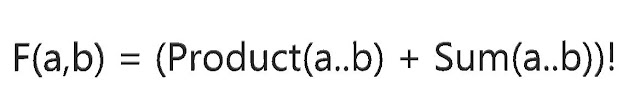## Posts

Showing posts from January, 2018

### LeetCode Hard Problem: Basic Calculator IIIProblem is here:  https://leetcode.com/problems/basic-calculator-iii/description/ Implement a basic calculator to evaluate a simple expression string. The expression string may contain open  (  and closing parentheses  ) , the plus  +  or minus sign  - ,  non-negative  integers and empty spaces  . The expression string contains only non-negative integers,  + ,  - ,  * ,  /  operators , open  (  and closing parentheses  )  and empty spaces  . The integer division should truncate toward zero. You may assume that the given expression is always valid. Some examples: "1 + 1" = 2 " 6-4 / 2 " = 4 "2*(5+5*2)/3+(6/2+8)" = 21 "(2+6* 3+5- (3*14/7+2)*5)+3"=-12 Now given the number of submissions and acceptances, this is definitely not a super trivial problem: Now since I had some ExpressionEvaluation code hanging around, I reused the code with some few modifications: Keep it to basic operations only Remove spaces from the expression C

### Using Bing and Hackerrank to solve LeetcodeThis problem from Leetcode was a fun one 'cause I got to use the Bing + Hackerrank Partnership  to solve it. Here is the problem  https://leetcode.com/problems/prime-number-of-set-bits-in-binary-representation/description/ : Given two integers  L  and  R , find the count of numbers in the range  [L, R]  (inclusive) having a prime number of set bits in their binary representation. (Recall that the number of set bits an integer has is the number of  1 s present when written in binary. For example,  21  written in binary is  10101  which has 3 set bits. Also, 1 is not a prime.) Example 1: Input: L = 6, R = 10 Output: 4 Explanation: 6 -> 110 (2 set bits, 2 is prime) 7 -> 111 (3 set bits, 3 is prime) 9 -> 1001 (2 set bits , 2 is prime) 10->1010 (2 set bits , 2 is prime) Example 2: Input: L = 10, R = 15 Output: 5 Explanation: 10 -> 1010 (2 set bits, 2 is prime) 11 -> 1011 (3 set bits, 3 is prime) 12 -> 1100 (2 set bits, 2 is prime) 13 -> 1101

### Shortest Completing Word, by LeetCode

Problem is this:  https://leetcode.com/problems/shortest-completing-word/description/ : Find the minimum length word from a given dictionary  words , which has all the letters from the string  licensePlate . Such a word is said to  complete  the given string  licensePlate Here, for letters we ignore case. For example,  "P"  on the  licensePlate  still matches  "p"  on the word. It is guaranteed an answer exists. If there are multiple answers, return the one that occurs first in the array. The license plate might have the same letter occurring multiple times. For example, given a  licensePlate  of  "PP" , the word  "pair" does not complete the  licensePlate , but the word  "supper"  does. Example 1: Input: licensePlate = "1s3 PSt", words = ["step", "steps", "stripe", "stepple"] Output: "steps" Explanation: The smallest length word that contains the letters "

### Messing around with infinity IIYears ago while messing around with infinity  there were several weird results that came up. Now check this out, we'll define the following mathematical formula that takes in two numbers "a" and "b" (assume that b>=a for simplicity), here it is: So function "F" takes two numbers a and b. First, it finds out the products of all the numbers from a to b. Then it finds out the sum of all the numbers from a to b. Adds them up. Takes the factorial of the result. One can easily implement this function, like this implementation below (C#):     class Program     { static void Main(string[] args) { long a = Int64.Parse(args); long b = Int64.Parse(args); Console.WriteLine("F({0},{1}) = {2}", a, b, Function(a, b)); } static long Factorial(long n) { long ret = 1; for (long i = 2; i <= n; i++) ret *= i; return ret; } static long Function(long a, long b) { long product = 1;

### Building a Full-Stack AI-Powered Feature on BingWhen you search the web you usually see a bunch of links in the results, you click on one of them, and quickly forget about that search engine. But behind those blue links and media content, there is some deep science, engineering and mathematics that take place. In this post I'll explain the high-level process (well, there will be some details) to build a very small feature, but you'll see how much effort and science go into it. Not rocket science, but gets very close to it. At Bing   (in particular the Visual System and SERP Experiences group) we've recently shipped a cool feature for mobile devices: say that you're searching on Google for a specific app: "1 second everyday app" (the current number #1 free app in the app store). This is what you get: As you can see the very first result is what you're looking for. Job well done. When you search the same query on Bing, however, this is what you get: You still get the same result as Google